# MATLAB Assignment 2

## Math 222 Spring 2021

### Question 1:

Apply the Euler method to solvefrom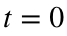to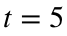.
Note that this problem has exact solution.
It is also easy to see how badly the method fails when you take N too large.
Then do the following:
• Figure out what is the minimum value of N that you need to get an error smaller than 0.01 at the final time. Do this by experimenting with N until you get a small enough error.
• Plot the computed and exact solution using this value of N.
I found that 1062 points was enough, but that 1061 was not. That's a lot!
tFinal = 5;
y0=1;
N = 1062;
h=tFinal/N;
t=linspace(0,tFinal,N+1); % type 'help linspace' to see how this works
y=zeros(1,N+1);y(1)=y0;
yX=yExact(t);
for n=1:N
y(n+1) = y(n) + h * f(t(n),y(n));
end
plot(t,y,t,yX,'--'); xlabel('t'); ylabel('y');
title('Result from Euler''s method');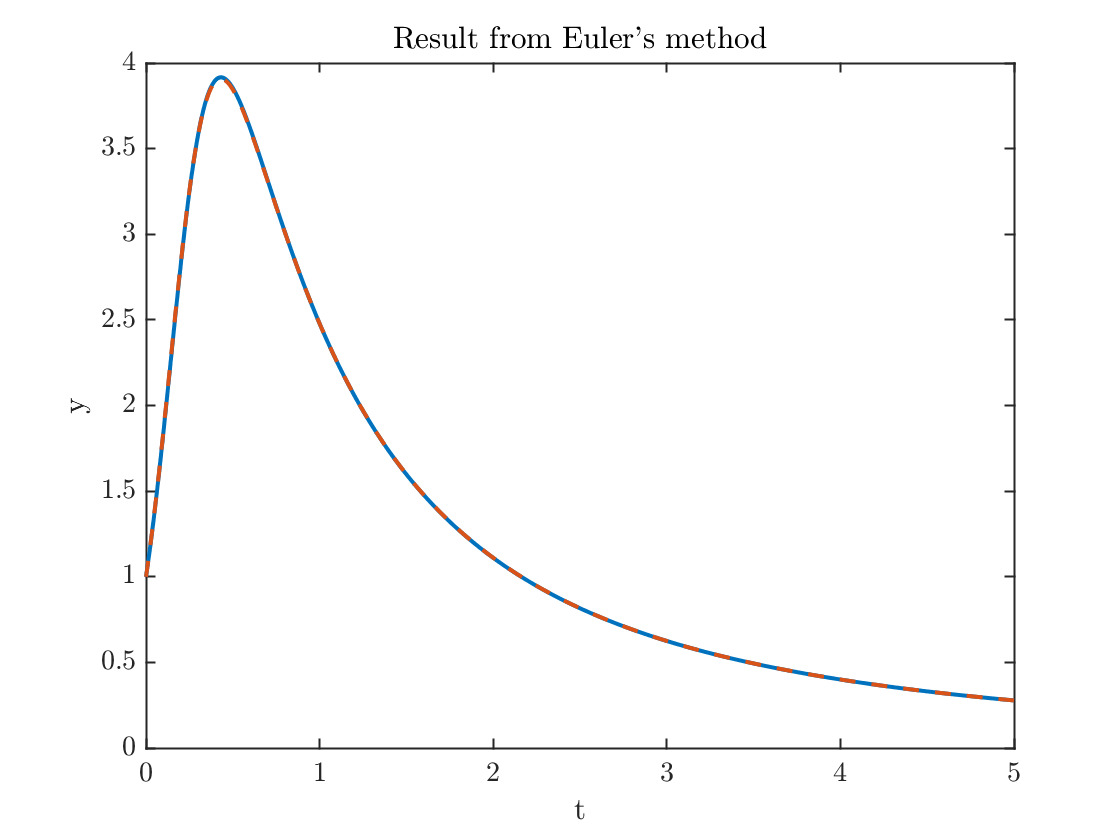plot(t,yX-y);xlabel('t');ylabel('error')
title('error from Euler''s method')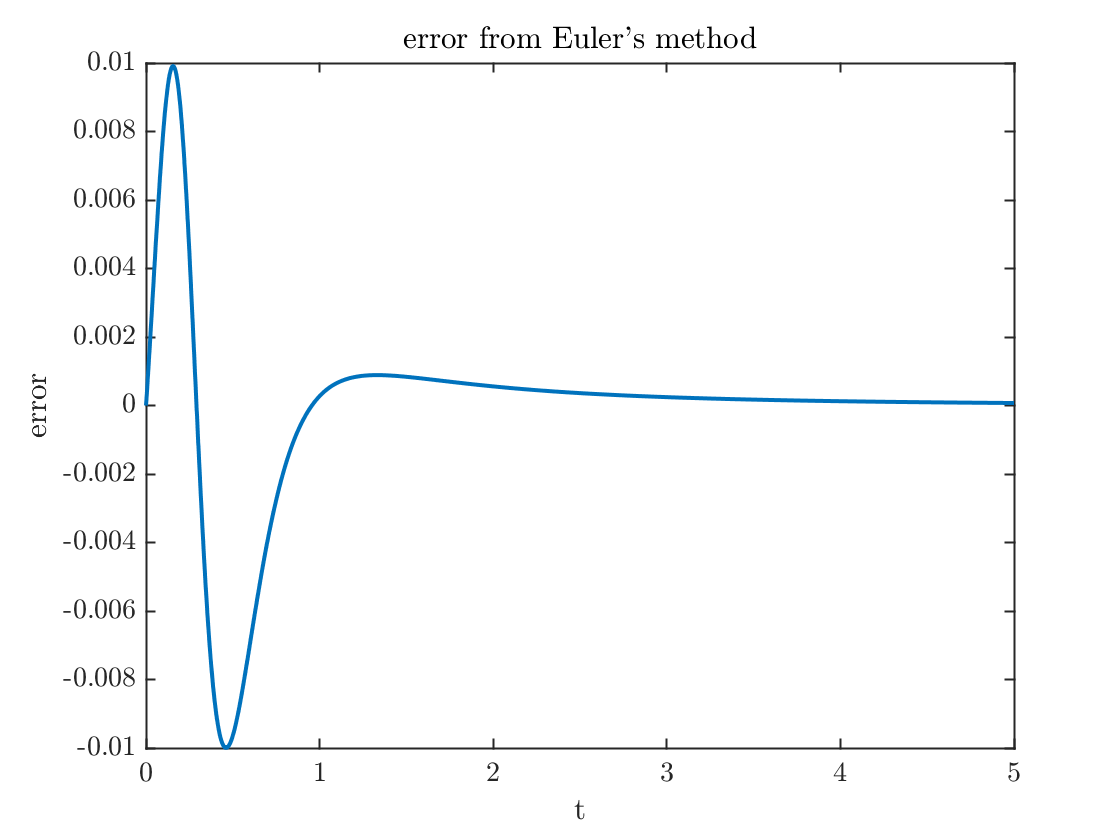error1= max(abs(y-yX));
fprintf('The maximum computed error is %0.4f.\n',error1)
The maximum computed error is 0.0100.
• Show the method is approximately second order by doubling N and computing the ratio of the errors.
N = 2*N;
h=tFinal/N;
t=linspace(0,tFinal,N+1); % type 'help linspace' to see how this works
y=zeros(1,N+1); y(1)=y0;
yX=yExact(t);
for n=1:N
y(n+1) = y(n) + h * f(t(n),y(n));
end
error2= max(abs(y-yX));
fprintf('The maximum error is %0.4f.\n',error2)
The maximum error is 0.0050.
fprintf('The error went down by a factor of %0.2f.\n',error1/error2);
The error went down by a factor of 2.00.

### Question 2

What is the minimum value of N you need to get the maximum error below 0.01?
I found I needed N=175.
N = 175;
h=tFinal/N;
t=linspace(0,tFinal,N+1); % type 'help linspace' to see how this works
y=zeros(1,N+1);y(1)=y0;
yX=yExact(t);
for n=1:N
k1=f(t(n),y(n));
k2=f(t(n)+h,y(n)+h*k1);
y(n+1) = y(n) + h * (k1+k2)/2;
end
plot(t,y,t,yX,'--'); xlabel('t'); ylabel('y');
title('Result from Improved Euler''s method');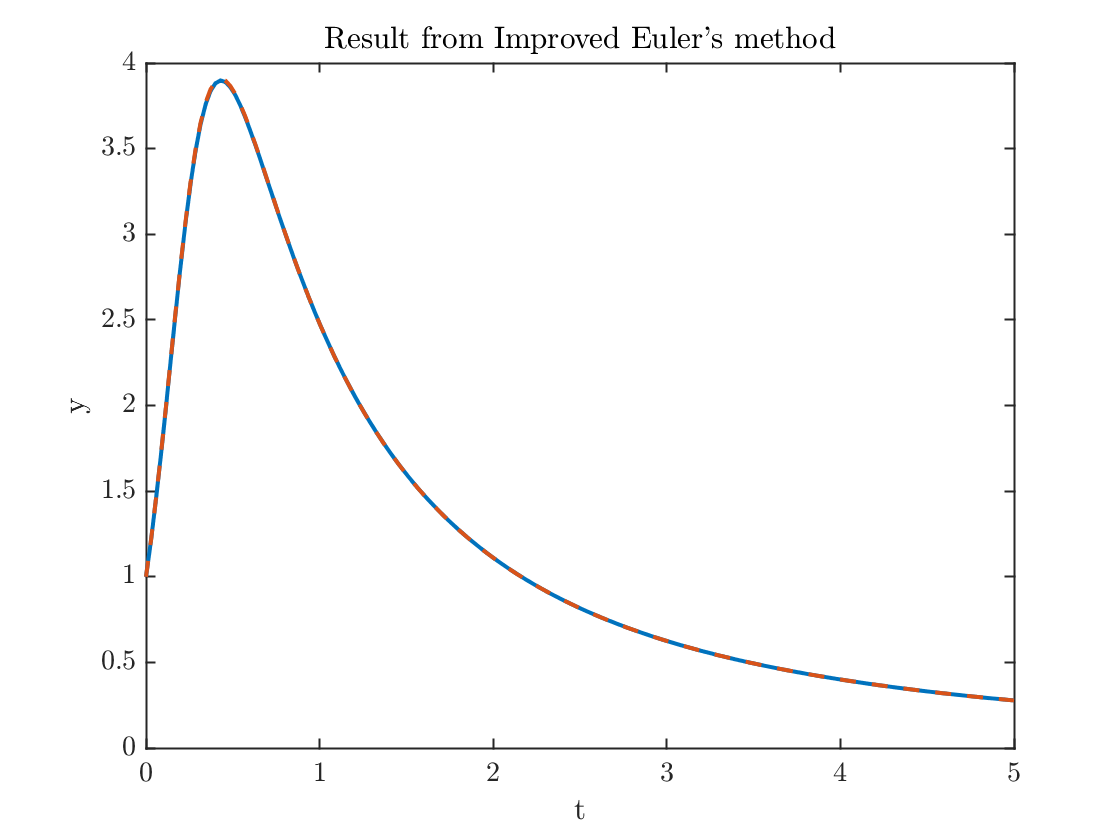plot(t,yX-y);xlabel('t');ylabel('error')
title('Error from Improved Euler''s method')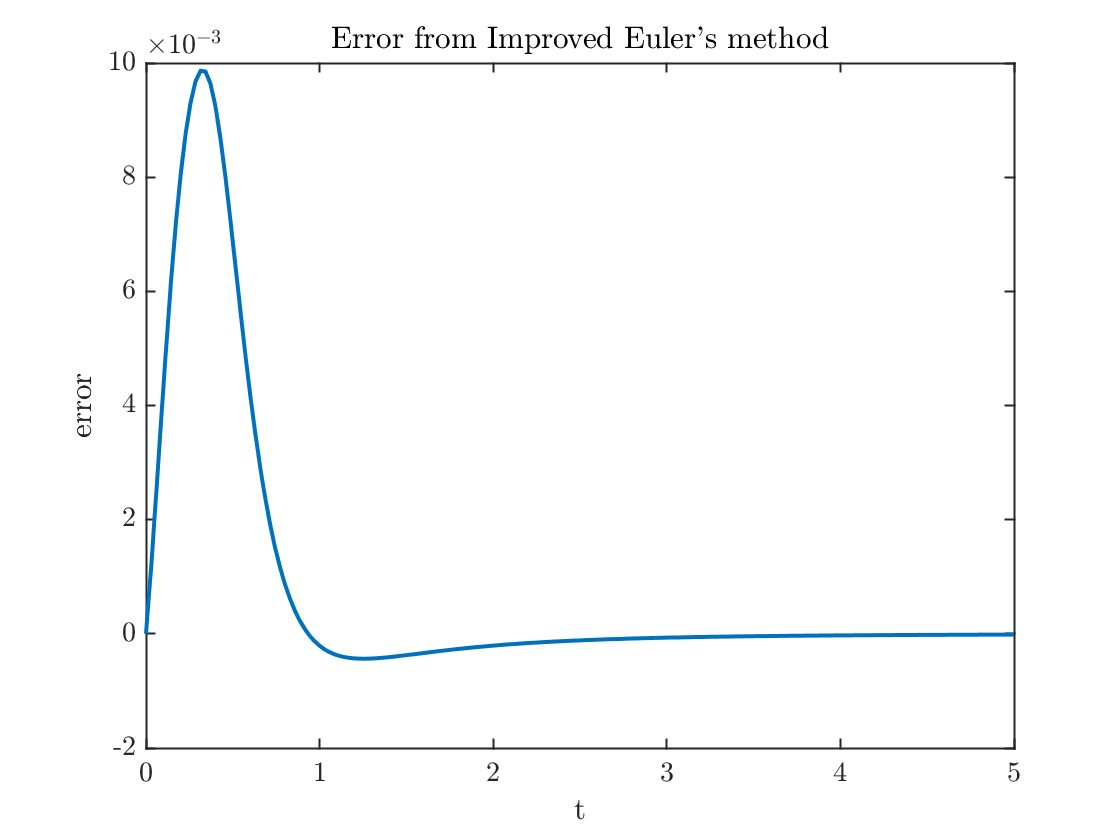error1= max(abs(y-yX));
fprintf('The maximum error is %0.4f.\n',error1)
The maximum error is 0.0099.
Now we double the number of points and see the error goes down by a factor of four.
N = 2*N;
h=tFinal/N;
t=linspace(0,tFinal,N+1); % type 'help linspace' to see how this works
y=zeros(1,N+1); y(1)=y0;
yX=yExact(t);
for n=1:N
k1=f(t(n),y(n));
k2=f(t(n)+h,y(n)+h*k1);
y(n+1) = y(n) + h * (k1+k2)/2;
end
error2= max(abs(y-yX));
fprintf('The maximum error is %0.df.\n',error2)
The maximum error is 2e-03f.
fprintf('The error went down by a factor of %0.2f.\n',error1/error2);
The error went down by a factor of 3.99.

## Functions used

function yprime=f(t,y)
yprime = 8*y/(t+1)-(t+1)*y^2;
end
function y=yExact(t)
y=10*(t+1).^8./((t+1).^10+9);
end